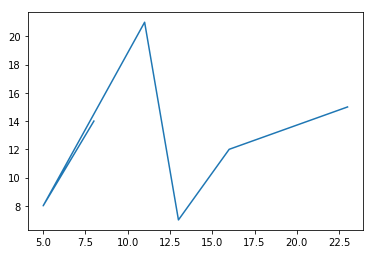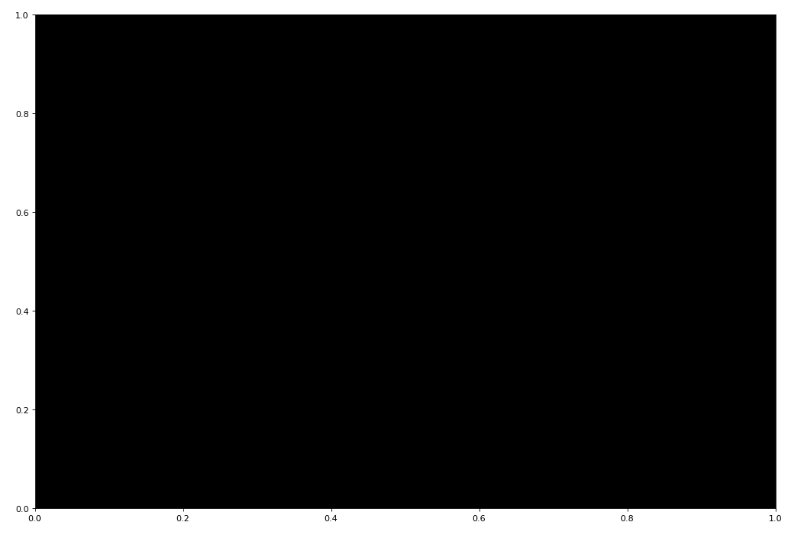Related Articles

# Matplotlib.pyplot.axes() in Python

• Last Updated : 17 May, 2020

Pyplot is another module of matplotlib that enables users to integrate MATLAB within Python environment, and thus providing MATLAB like interface and making Python visually interactive.

## Matplotlib.pyplot.axes()

`pyplot.axes` is a function of the matplotlib library that adds axes to the current graph and makes it as current axes. Its output depends on the arguments used.

Attention geek! Strengthen your foundations with the Python Programming Foundation Course and learn the basics.

To begin with, your interview preparations Enhance your Data Structures concepts with the Python DS Course. And to begin with your Machine Learning Journey, join the Machine Learning - Basic Level Course

Syntax: matplotlib.pyplot.axes(*args, **kwargs)

Parameters:
*args: It may include either None(nothing) or 4 tuples of float type

• none: It gives a new full window axes
• 4 tuples: It takes 4 tuples as list i.e [left bottom width height] and gives a window of these dimensions as axes.

**kwargs: There are several keyword arguments(kwargs) used as parameters to pyplot.axes(), most common include facecolor, gid, in_layout, label, position, xlim, ylim, etc.

Example 1:

 `# importing matplot library along ``# with necessary modules``import` `matplotlib.pyplot as plt`` ` ` ` `# providing values to x and y ``x ``=` `[``8``, ``5``, ``11``, ``13``, ``16``, ``23``]``y ``=` `[``14``, ``8``, ``21``, ``7``, ``12``, ``15``]`` ` `# to plot x and y``plt.plot(x, y)`` ` `# to generate the full window axes``plt.axes()`

Output:Example 2:

 `# importing matplot library along ``# with necessary modules``import` `matplotlib.pyplot as plt`` ` ` ` `# providing values to x and y ``x ``=` `[``8``, ``5``, ``11``, ``13``, ``16``, ``23``]``y ``=` `[``14``, ``8``, ``21``, ``7``, ``12``, ``15``]`` ` `# to plot x and y``#plt.plot(x, y)``# to generate window of custom ``# dimensions [left, bottom, width,``# height] along with the facecolor ``plt.axes([``0``, ``2.0``, ``2.0``, ``2.0``], facecolor ``=` `'black'``) `

Output:My Personal Notes arrow_drop_up